Function Repository Resource:

# LogarithmicNorm

Evaluate the logarithmic norm of a square matrix

Contributed by: Jan Mangaldan
 ResourceFunction["LogarithmicNorm"][m,p] gives the logarithmic p‐norm of the matrix m.

## Details

m must be a square matrix.
p can be any of 1, 2 or .
The logarithmic norm μp(m) is defined as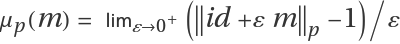, where id is the identity matrix of appropriate size and the norm satisfies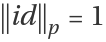.

## Examples

### Basic Examples (3)

Logarithmic 1-norm of a 3×3 matrix:

 In:=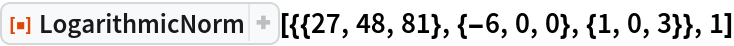Out=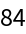Logarithmic -norm of a 3×3 matrix:

 In:=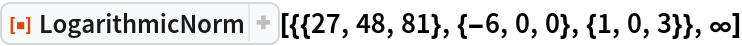Out=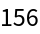Logarithmic 2-norm of a 3×3 matrix:

 In:=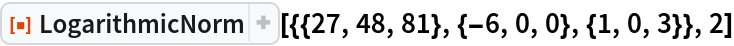Out=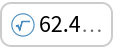### Scope (2)

A 3×3 matrix:

 In:=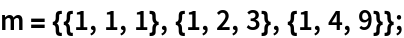Evaluate the logarithmic norm with exact arithmetic:

 In:=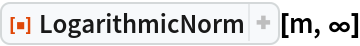Out=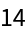Evaluate the logarithmic norm with machine arithmetic:

 In:=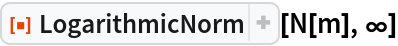Out=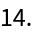Evaluate the logarithmic norm with 20­digit arbitrary precision arithmetic:

 In:=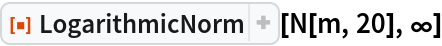Out=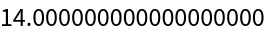Logarithmic norm of a sparse matrix:

 In:=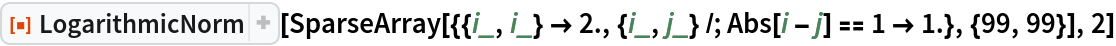Out=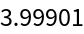### Properties and Relations (2)

The logarithmic norm can be negative, and is thus not a matrix norm:

 In:=Out=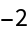The logarithmic 2-norm of m is equal to the largest eigenvalue of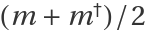:

 In:=Out=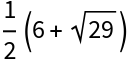In:=Out=## Version History

• 1.0.0 – 19 January 2022